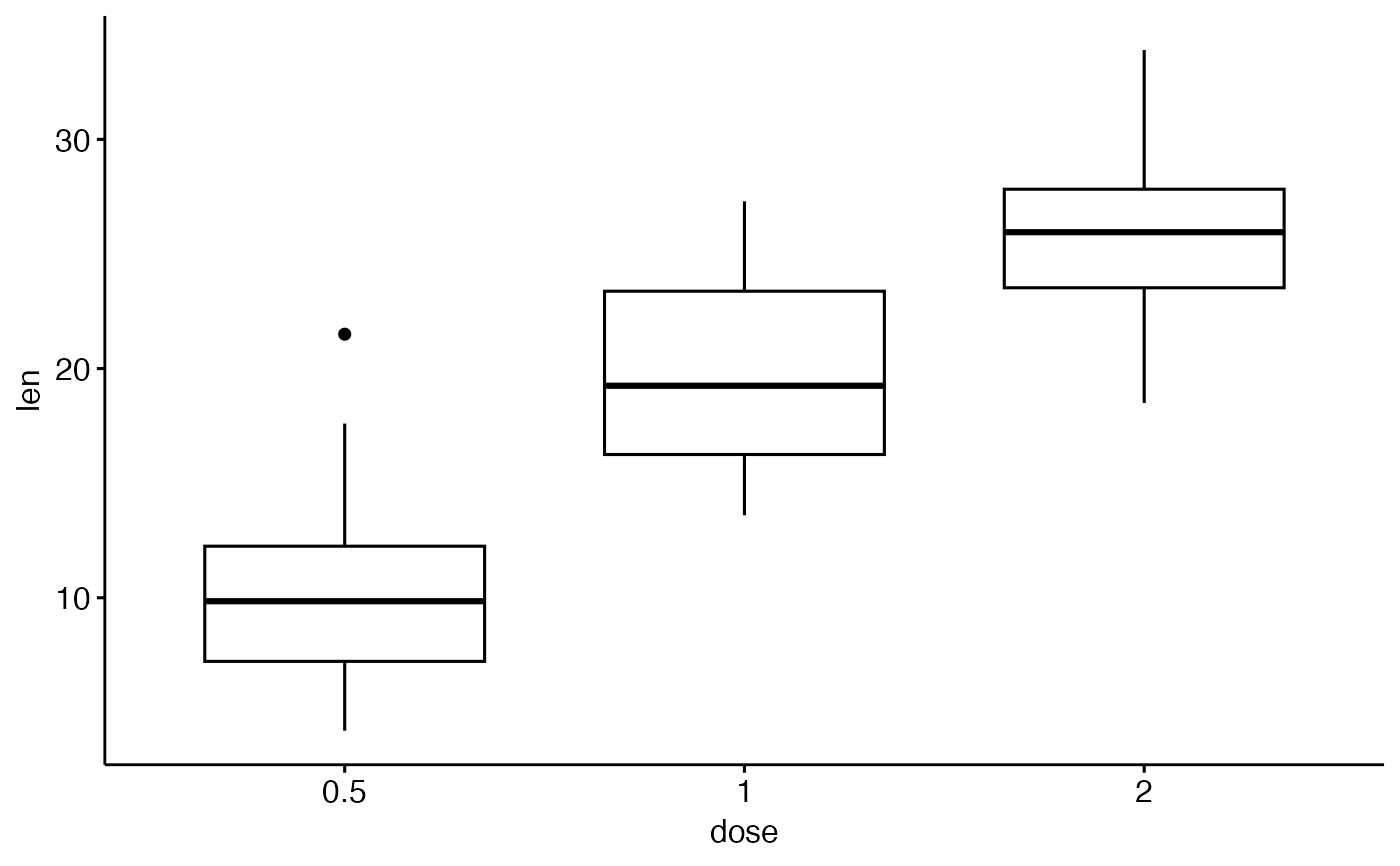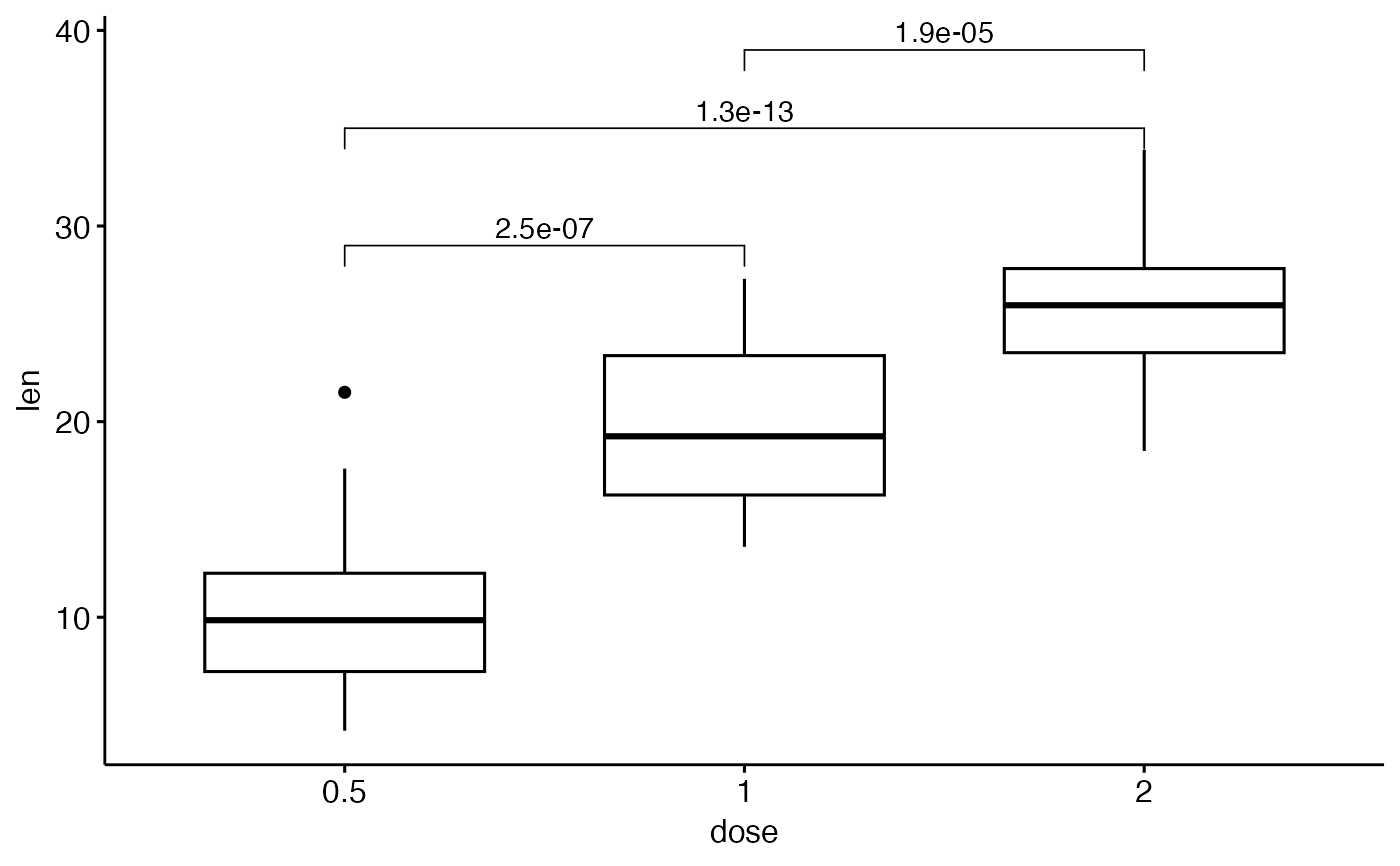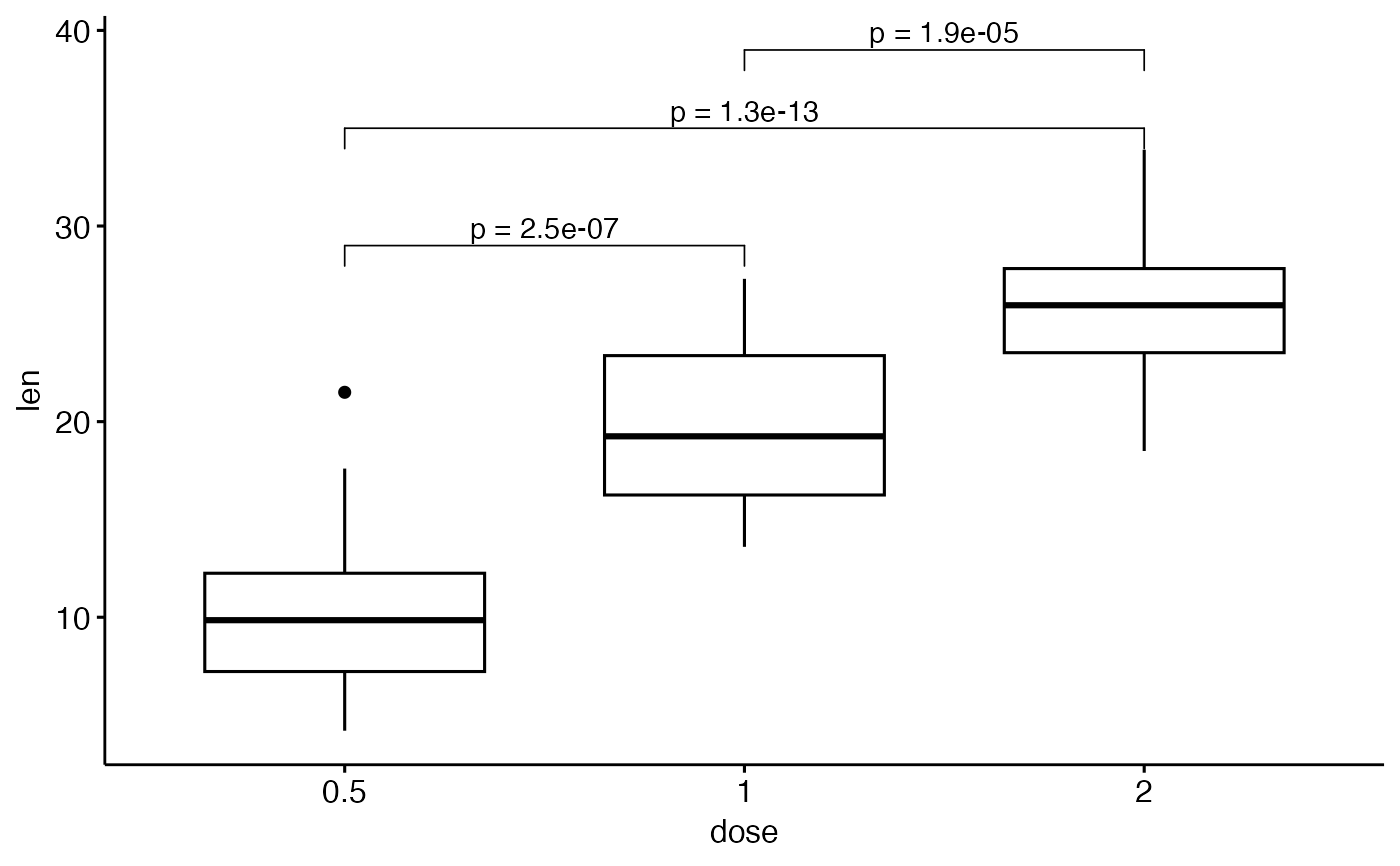Add manually p-values to a ggplot, such as box blots, dot plots and stripcharts. Frequently asked questions are available on Datanovia ggpubr FAQ page, for example:

stat_pvalue_manual(
data,
label = NULL,
y.position = "y.position",
xmin = "group1",
xmax = "group2",
x = NULL,
size = 3.88,
label.size = size,
bracket.size = 0.3,
bracket.nudge.y = 0,
bracket.shorten = 0,
color = "black",
linetype = 1,
tip.length = 0.03,
remove.bracket = FALSE,
step.increase = 0,
step.group.by = NULL,
hide.ns = FALSE,
vjust = 0,
coord.flip = FALSE,
position = "identity",
...
)

## Arguments

data

a data frame containing statitistical test results. The expected default format should contain the following columns: group1 | group2 | p | y.position | etc. group1 and group2 are the groups that have been compared. p is the resulting p-value. y.position is the y coordinates of the p-values in the plot.

label

the column containing the label (e.g.: label = "p" or label = "p.adj"), where p is the p-value. Can be also an expression that can be formatted by the glue() package. For example, when specifying label = "t-test, p = {p}", the expression {p} will be replaced by its value.

y.position

column containing the coordinates (in data units) to be used for absolute positioning of the label. Default value is "y.position". Can be also a numeric vector.

xmin

column containing the position of the left sides of the brackets. Default value is "group1".

xmax

(optional) column containing the position of the right sides of the brackets. Default value is "group2". If NULL, the p-values are plotted as a simple text.

x

x position of the p-value. Should be used only when you want plot the p-value as text (without brackets).

size, label.size

size of label text.

bracket.size

Width of the lines of the bracket.

bracket.nudge.y

Vertical adjustment to nudge brackets by. Useful to move up or move down the bracket. If positive value, brackets will be moved up; if negative value, brackets are moved down.

bracket.shorten

a small numeric value in [0-1] for shortening the with of bracket.

color

text and line color. Can be variable name in the data for coloring by groups.

linetype

linetype. Can be variable name in the data for changing linetype by groups.

tip.length

numeric vector with the fraction of total height that the bar goes down to indicate the precise column. Default is 0.03.

remove.bracket

logical, if TRUE, brackets are removed from the plot. Considered only in the situation, where comparisons are performed against reference group or against "all".

step.increase

numeric vector with the increase in fraction of total height for every additional comparison to minimize overlap.

step.group.by

a variable name for grouping brackets before adding step.increase. Useful to group bracket by facet panel.

hide.ns

can be logical value or a character vector.

• Case when logical value. If TRUE, hide ns symbol when displaying significance levels. Filter is done by checking the column p.adj.signif, p.signif, p.adj and p.

• Case when character value. Possible values are "p" or "p.adj", for filtering out non significant.

vjust

move the text up or down relative to the bracket. Can be also a column name available in the data.

coord.flip

logical. If TRUE, flip x and y coordinates so that horizontal becomes vertical, and vertical, horizontal. When adding the p-values to a horizontal ggplot (generated using coord_flip()), you need to specify the option coord.flip = TRUE.

position

position adjustment, either as a string, or the result of a call to a position adjustment function.

...

other arguments passed to the function geom_bracket() or geom_text()

stat_compare_means

## Examples


# T-test
stat.test <- compare_means(
len ~ dose, data = ToothGrowth,
method = "t.test"
)
stat.test
#> # A tibble: 3 × 8
#>   .y.   group1 group2        p   p.adj p.format p.signif method
#>   <chr> <chr>  <chr>     <dbl>   <dbl> <chr>    <chr>    <chr>
#> 1 len   0.5    1      1.27e- 7 2.5e- 7 1.3e-07  ****     T-test
#> 2 len   0.5    2      4.40e-14 1.3e-13 4.4e-14  ****     T-test
#> 3 len   1      2      1.91e- 5 1.9e- 5 1.9e-05  ****     T-test

# Create a simple box plot
p <- ggboxplot(ToothGrowth, x = "dose", y = "len")
p# Perform a t-test between groups
stat.test <- compare_means(
len ~ dose, data = ToothGrowth,
method = "t.test"
)
stat.test
#> # A tibble: 3 × 8
#>   .y.   group1 group2        p   p.adj p.format p.signif method
#>   <chr> <chr>  <chr>     <dbl>   <dbl> <chr>    <chr>    <chr>
#> 1 len   0.5    1      1.27e- 7 2.5e- 7 1.3e-07  ****     T-test
#> 2 len   0.5    2      4.40e-14 1.3e-13 4.4e-14  ****     T-test
#> 3 len   1      2      1.91e- 5 1.9e- 5 1.9e-05  ****     T-test

# Add manually p-values from stat.test data
# First specify the y.position of each comparison
stat.test <- stat.test %>%
mutate(y.position = c(29, 35, 39))
p + stat_pvalue_manual(stat.test, label = "p.adj")# Customize the label with glue expression
# (https://github.com/tidyverse/glue)
p + stat_pvalue_manual(stat.test, label = "p = {p.adj}")# Grouped bar plots
#%%%%%%%%%%%%%%%%%%%%%%%%%%%%%%%%%%%%%%%%%%%
ToothGrowth$dose <- as.factor(ToothGrowth$dose)
# Comparisons against reference
stat.test <- compare_means(
len ~ dose, data = ToothGrowth, group.by = "supp",
method = "t.test", ref.group = "0.5"
)
stat.test
#> # A tibble: 4 × 9
#>   supp  .y.   group1 group2            p      p.adj p.format p.signif method
#>   <fct> <chr> <chr>  <chr>         <dbl>      <dbl> <chr>    <chr>    <chr>
#> 1 VC    len   0.5    1      0.000000681  0.000002   6.8e-07  ****     T-test
#> 2 VC    len   0.5    2      0.0000000468 0.00000019 4.7e-08  ****     T-test
#> 3 OJ    len   0.5    1      0.0000878    0.000088   8.8e-05  ****     T-test
#> 4 OJ    len   0.5    2      0.00000132   0.0000026  1.3e-06  ****     T-test
# Plot
bp <- ggbarplot(ToothGrowth, x = "supp", y = "len",
fill = "dose", palette = "jco",